## Each year the admissions committee at a top business school receives a large number of applications for admission to the MBA program and the

Question

in progress 0
2 months 2021-08-21T22:52:35+00:00 1 Answers 7 views 0

0.1711 = 17.11% probability that the class size will be at least 720 students

Step-by-step explanation:

Normal Probability Distribution:

Problems of normal distributions can be solved using the z-score formula.

In a set with mean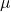and standard deviation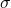, the z-score of a measure X is given by: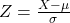The Z-score measures how many standard deviations the measure is from the mean. After finding the Z-score, we look at the z-score table and find the p-value associated with this z-score. This p-value is the probability that the value of the measure is smaller than X, that is, the percentile of X. Subtracting 1 by the p-value, we get the probability that the value of the measure is greater than X.

In this question:

Our random variable X is the number of students not taking admission, which has mean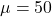and standard deviation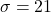a. Suppose 750 students are admitted. What is the probability that the class size will be at least 720 students?

30 or less students do not take admission, which means that this is the pvalue of Z when X = 30.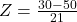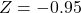has a pvalue of -0.1711

0.1711 = 17.11% probability that the class size will be at least 720 students